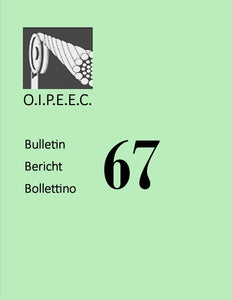# Endurance of steel wire ropes in tree bending conditions

• €0,00

The objective of the project described in this article was to find a prediction formula for the endurance of wire ropes in free bending conditions. This free bending prediction formula is in the form of the ""Feyrer'' method. For the use of the derived formula coefficients had to be determined. The determination of these coefficients was by a linear regression based on data from test results. A validation of the formula has been made by two additional tests, as well as with the results of the tests the coefficients were based on. From this validation the conclusion can be drawn that the predictions are fairly accurate and that the derived formula can be used for the prediction of the endurance of the rope in free bending conditions. However, more tests and better analysis of the results are necessary before the formula can be used for practical applications.

Author: B.G. Van der Sterren